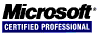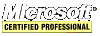# JavaScript Tutorial

## Operators

Operators are used to manipulate data values.

### Unary Operators

Unary operators work on only one value.

#### Increment/Decrement

Increment and decrement operators come in two flavors: prefix and postfix. These operators only work on variables. The prefix one works as one would expect.

``````var num = 10;
var i;
i = ++num + 10; // i is 21, num is 11;
i = --num + 10; // i is 20, num is 10;``````

The postfix increment/decrement operators are not so obvious.

``````var num = 10;
var i;
i = num++ + 10; // i is 20, num is 11;
i = num-- + 10; // i is 21, num is 10;``````

The best way to understand this behavior is to see it as `num` being incremented/decremented after the statement.

These operators can be used on any datatype. They will convert strings to numbers and then add/subtract them by one. If the string can not be converted to a number then they are converted to `NaN`. `false` and `true` are converted to 0 and 1, respectively, and the variable is converted to a number. When used on a floating point values, one is added/subtracted. When used on an object, the `valueOf()` method is called; the rules just mentioned are applied unless the result is `NaN` then call `toString()` and once again apply the rules just mentioned. The variable is changed from an object to a number.

``````var f = 2.1;
var b = true;
var s1 = "2";
var s2 = "z";
var o = {
valueOf: function() {
return 0;
}
toString: function() {
return "0";
}
};
f--;	// the value is 1.1
b++;	// the value is numeric 2
s1++; // the value is numeric 3
s2++; // the value is NaN
o++;	// the value is numeric 1``````

#### Unary Plus and Minus

The unary plus is a single plus sign (+) placed before a variable and does nothing to a numeric value. When the unary plus is applied to a nonnumeric value, it performs the same conversion as the `Number()` casting function.

The unary minus is a single negative sign (-) placed before a variable to negate it. For example, converting 1 to -1. It has all the same conversion rules as unary plus.

<< < [Page 4 of 22] > >>